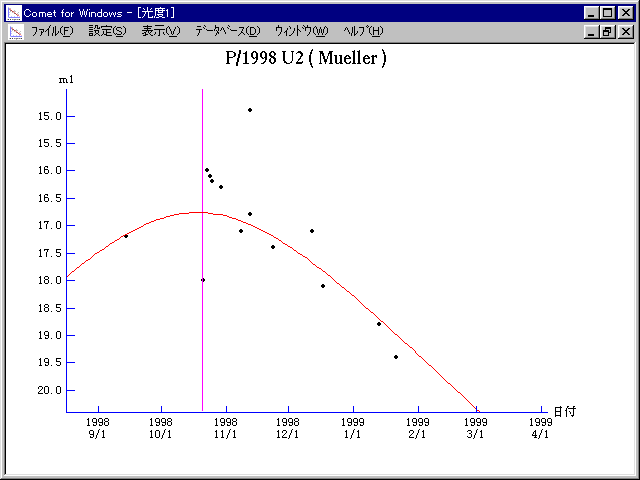# \$B%_%e%i!

190P/Mueller (1998)###\$B%W%m%U%#!<%k(B

 \$BId9f(B 190P/1998 U2 \$BH/8+F|(B 1998\$BG/(B10\$B7n(B21\$BF|(B \$BH/8+8wEY(B 18\$BEy(B \$BH/8+ J. Mueller (Palomar Outer Solar System Ecliptic Survey)

###\$B###\$B50F;MWAG(B

```                    Epoch = 1998 Nov.  3.0 TT
T = 1998 Oct. 20.75954 TT        Peri. =  49.53569
e = 0.5215660                    Node  = 336.22405 2000.0
q = 2.0253092 AU                 Incl. =   2.19329
a =  4.2332052 AU   n = 0.11316187   P =   8.71 years
```

###\$B@1?^(B###\$B8wEYJQ2=(B

```        m1 = 7.5 + 5 log\$B&\$(B + 30.0 log r
```##### \$B50F;MWAG\$O!"(B IAUC 8859 \$B\$K7G:\\$5\$l\$?\$b\$N\$G\$9!#(B \$B@1?^\$O(B StellaNavigator Ver.2.0 for Windows (\$B%"%9%H%m%"!<%D(B \$BJTCx(B / \$B%"%9%-!<=PHG6I4)(B) \$B\$G:n@.\$7\$?\$b\$N\$G\$9!#(B \$B8wEY%0%i%U\$O(BComet for Windows\$B\$G:n@.\$7\$?\$b\$N\$G\$9!#(B